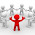## Monday, April 4, 2016

### SQL Tutorial In Urdu - TSQL Case Statement

```-- Example 1
SELECT StudentId, Name, Email, Mobile,
CASE RegistrationType
WHEN 1 THEN 'Online'
WHEN 2 THEN 'In Person'
WHEN 3 THEN 'By Phone'
WHEN 4 THEN 'Email'
WHEN 5 THEN 'By Post'
END AS 'Registration Type'
, DateOfRegistration, Gender
FROM [dbo].[Students]

-- Example 2
SELECT ResultId,
StudentId,
ReleaseDate,
ExamTotal,
StudentTotal
, CASE WHEN [StudentTotal] < 300 THEN 'Fail' ELSE 'Pass' END AS Result
, CASE
WHEN ([StudentTotal] * 100.0 /[ExamTotal]) > 80 THEN 'A1'
WHEN ([StudentTotal] * 100.0 /[ExamTotal]) > 70 THEN 'A'
WHEN ([StudentTotal] * 100.0 /[ExamTotal]) > 60 THEN 'B'
WHEN ([StudentTotal] * 100.0 /[ExamTotal]) > 50 THEN 'C'
WHEN ([StudentTotal] * 100.0 /[ExamTotal]) >= 40 THEN 'D'
WHEN ([StudentTotal] * 100.0 /[ExamTotal]) < 40 THEN 'F'
FROM [dbo].[StudentResults]
```

1.1.